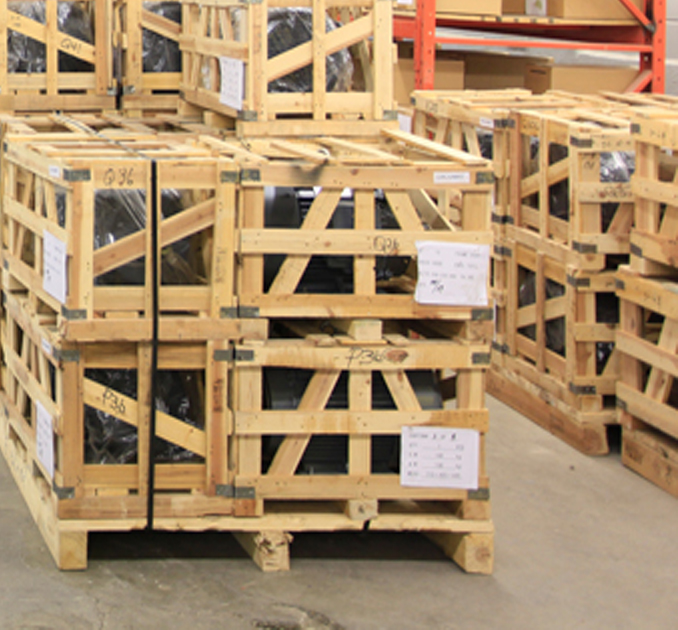# What Does Gear Reduction (Gearbox) actually mean for DC gearbox motors?

Thursday - 22/11/2012 16:52
DC Gear motor,is also called DC Geared Motor/Geared DC Motor/Gearhead motor or Gearbox motor.
It consists of a electric DC motor and gear reduction;these gearboxes are used to reduce the DC motor speed while increase the DC motor torque as well.Therefore lower speed and higher torque solution will be obtained from gear motor accordingly.

On the surface, it may seem that gears are being “reduced” in quantity or size which is actually partially true.When an engine needs the output speed reduced and/or torque increased,gears are commonly used to accomplish the desired result.

Gear“reduction” specifically refers to the speed of the motor;the rotational speed of the motor is “reduced” by dividing it by a gear ratio greater than 1:1. A gear ratio greater than 1:1 is achieved when a smaller gear (reduced size) with fewer number of teeth meshes and drives a larger gear with greater number of teeth.Gear reduction has the opposite effect on torque.The dc motor’s output torque is increased by multiplying the torque by the gear ratio, less some efficiency losses.

While in many applications gear reduction reduces speed and increases torque,in other applications gear reduction is used to increase speed and reduce torque.

Generators in wind turbines use gear reduction in this manner to convert a relatively slow turbine blade speed to a high speed capable of generating electricity.These applications use gearboxes that are assembled opposite of those in applications that reduce speed and increase torque.

How is gear reduction achieved? Many reducer types are capable of attaining gear reduction including,but not limited to, parallel shaft (Spur gearbox), planetary and right-angle worm gearboxes.In parallel shaft gearbox(or reducers),a pinion gear with a certain number of teeth meshes and drives a larger gear with a greater number of teeth. The “reduction” or gear ratio is calculated by dividing the number of teeth on the large gear by the number of teeth on the small gear.

For example, if an electric motor drives a 13-tooth pinion gear that meshes with a 65-tooth gear, a reduction of 5:1 is achieved (65 / 13 = 5). If the electric motor speed is 3,450 rpm, the gearbox reduces this speed by five times to 690 rpm.

If the motor torque is 10kgf.cm, the gearbox increases this torque by a factor of five to 50kgf.cm (before subtracting out gearbox efficiency losses).

Parallel shaft gearboxes many times contain multiple gear sets thereby increasing the gear reduction. The total gear reduction (ratio) is determined by multiplying each individual gear ratio from each gear set stage.If a gearbox contains 3:1, 4:1 and 5:1 gear sets, the total ratio is 60:1 (3 x 4 x 5 = 60). In our example above, the 3,450 rpm electric motor would have its speed reduced to 57.5 rpm by using a 60:1 gearbox.

The 10kgf.cm electric motor torque would be increased to 600kgf.cm (before efficiency losses).

If a pinion gear and its mating gear have the same number of teeth, no reduction occurs and the gear ratio is 1:1. The gear is called an idler and its primary function is to change the direction of rotation rather than decrease the speed or increase the torque.

Calculating the gear ratio in a planetary gear reducer is less intuitive as it is dependent on the number of teeth of the sun and ring gears. The planet gears act as idlers and do not affect the gear ratio.

The planetary gear ratio equals the sum of the number of teeth on the sun and ring gear divided by the number of teeth on the sun gear.

For example, a planetary set with a 12-tooth sun gear and 72-tooth ring gear has a gear ratio of 7:1 ([12 + 72]/12 = 7). Planetary gear sets can achieve ratios from about 3:1 to about 11:1. If more gear reduction is needed, additional planetary stages can be used.The gear reduction in a worm driveis dependent on the number of threads or “starts” on the worm and the number of teeth on the mating worm wheel. If the worm has two starts and the mating worm wheel has 50 teeth, the resulting gear ratio is 25:1 (50 / 2 = 25).

When a motor cannot provide the desired output speed or torque, a gear reducer may provide a good solution. Parallel shaft (spur gearbox), planetary, right-angle worm drives are common gearbox types for achieving gear reduction.